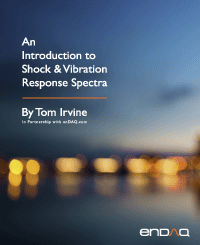Dynamic Models for External Excitation
By Tom Irvine
SECTIONS
SECTION 1

# Two Models

There are two basic SDOF spring-mass models used for external excitation in shock and vibration analysis. One is the applied force model, and the other is the base excitation model. An introduction to these types and images was previously given. The purpose of this section is to demonstrate solution methods for each of these type in both the time and frequency domains.

SECTION 2

## Applied Force

SDOF Response Equations

The applied force model and its free-body diagram are

The equation of motion for applied force F is derived using Newton’s law.

The resulting equation of motion is non-homogenous. It can be solved via Laplace transforms if the force is deterministic such as a sine function. A convolution integral is needed if the force varies arbitrary with time. The resulting displacement for an applied force is

The convolution integral in equation (1.5) can be solved in terms of a ramp invariant digital recursive relationship .

Alternate methods for numerically solving equation (1.4) include the Runge-Kutta and Newmark-beta methods. The Runge-Kutta method may unstable for stiff systems, however.

The displacement convolution integral has an embedded impulse response function.

The Laplace transform of the impulse response function is the steady-state frequency response function (FRF).

This FRF can be represented in the frequency domain by setting s = jω, where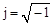.

The FRF in equation (1.8) is the Fourier transform of the impulse response function in equation (1.7). Fourier transforms are covered in Section 15. An equivalent FRF is

Input & Response Example, Applied Force

The single-degree-of-freedom system in Figure 12.1 has a mass of 20 kg, stiffness of 78960 N/m, natural frequency of 10 Hz and an amplification factor Q=10 for this example. It is excited into resonance by a 20 N applied force sinusoidal excitation at 10 Hz. The exact response can be calculated via a Laplace transform solution. The result is shown in Figure 1.2.

The peak response is 2.53 mm. Note that the following ratio is approximately equal to the Q value.

The response calculation could be repeated for numerous frequency and amplification factor cases to determine the maximum steady-state response for each case. The transfer function represents the displacement divided by the applied force as normalized by the stiffness such that the magnitude is unitless, as previously introduced. The transfer function magnitude is

The phase angle by which the displacement lags the force is

The applied force transfer function in Figure 1.3 can roughly be divided into three zones. The function is controlled by stiffness at the low frequency ratio end. Its magnitude converges to the simple static Hooke’s law as the frequency ratio approaches zero. The function is controlled by damping for frequency ratios at or near the resonance condition where the ratio is equal to 1. The function is controlled by mass at higher ratios, above √2.

Six Transfer Functions for Applied Force and Response

Recall equation (1.8). The following equation represents the ratio of displacement to force in terms of their respective Fourier transforms.

Similar frequency response ratios can be formed for the velocity and acceleration responses relative to force. Inverses of these functions can also be taken. The names of these transfer functions are given in the following tables.

Table 2.1. Transfer Function Nomenclature
Displacement / Force Velocity / Force Acceleration / Force
Compliance,
Receptance
Mobility Accelerance,
Inertance
Table 2.2. Reciprocal Transfer Function Nomenclature
Force / Displacement Force / Velocity Force / Acceleration
Dynamic Stiffness Mechanical Impedance Apparent Mass,
Dynamic Mass

Furthermore, the receptance frequency response function can be modeled in terms of a block diagram. Similar diagrams could be drawn for the other transfer functions.

Helicopter Ground Resonance

A sketch of a generic helicopter is shown in Figure 1.5. The helicopter may be modeled as a single-degree-of-freedom system excited by an applied force.

A new helicopter design must undergo thorough testing to ensure the reliability of the design with respect to vibration. It may encounter severe vibration while it is on the ground, preparing for takeoff. A similar problem may occur immediately after landing.

Many helicopters have fully-articulated rotor systems. The advancing blades flap upward and the retreating blades flap downward in order to maintain uniform lift during forward flight. A helicopter with a fully-articulated rotor has some measure of an unbalanced rotational force, which causes an oscillating force. This force may excite one of the helicopter’s natural frequencies, as the rotor accelerates to its full operating speed prior to takeoff. This condition is called resonant excitation.

John O. Emmerson wrote in Reference :

In the mid-1950s, the emerging helicopter introduced a frightening problem called ground resonance. A perfectly sound production helicopter firmly supported on its landing gear with the rotor turning at or near flight rpm would suddenly initiate a “tramping” on the gear and then, almost immediately, completely disintegrate. The Sikorsky company, which was leading the pack in developing the helicopter, quickly gathered the most data on these ground-based disasters and thus shouldered the lion’s share of corrective understanding and action. The company established that the lead/lag pivots on the rotor blades, the pylon elastic stiffness, and the elastic components of the landing gear, including the tire and shock struts, all working together as a single structural entity, had an unfortunate resonant frequency near the rotor operating rpm. The engineers were able to show that a fearless pilot, entering the resonant phase, could save the day be pulling up the collective pitch control and unloading the landing gear (i.e., getting airborne). Lowering the resonant frequency and adding damping offered a permanent solution.

Today, all of us in the helicopter development business, with the apprehension borne of substantial ignorance, approach initial ground whirl testing of each new helicopter with tremulous respect for the demon of ground resonances.

Modern helicopters avoid ground resonance by using dampers on the blades and on the landing gear. The landing gear may have shock absorbing struts.

An intact TH-55 helicopter is shown in Figure 1.6. A similar TH-55 helicopter was destroyed by ground resonance in March 1967, at Ft. Wolters, TX, as shown in Figure 1.7.

Two-Degree-of-Freedom System Subjected to Applied Forces
Two-Degree-of-Freedom System Equations of Motion, Time Domain

Recall the grounded, two-DOF system from Section 6.1.1. It is shown again in the above figure with added forces. The coupled equations of motion are

Note that each of the coefficient matrices is symmetric. This is typical for linear structural dynamics systems.

A shorthand form of equation (1.4) is

The matrices and vectors are

The natural frequencies and mode shapes are found from the undamped, homogeneous equation as was shown in Section 6.1.1. Now define a modal coordinate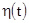in terms of the normalized eigenvector matrixsuch that the displacement vector is

Repeat the steps in Section 6.1.1 with the added force term.

The orthogonality relationships yield

The equation of motion can now be written with the modal damping simplification as

The two equations are now uncoupled in terms of the modal coordinates. Now consider the case where the forcing functions are deterministic time domain functions. The modal displacement for the response to the harmonic forces can be found via Laplace transforms. Alas, the resulting modal displacement equations even for sinusoidal forces are too unwieldy for inclusion in this book but are given in Reference . The physical displacements are then found via equation (1.17). The solution for the case of arbitrary forces can be calculated using the ramp invariant digital recursive filtering relationship in Reference .

Two-Degree-of-Freedom System, Time Domain Example

The system in Figure 12.18 has the parameters previously shown in Table 6.1, with 5% damping for each mode. Its natural frequencies are again 20.0 and 42.5 Hz. The mass-normalized mode shapes in matrix format are

It is subjected to a single sinusoidal force applied to the top mass, with zero initial conditions. The amplitude is 1 lbf. The forcing frequency is 20.0 Hz, which is the same as the fundamental frequency. The results are shown in Figure 12.9.

Now consider the steady-state amplitude ratios between the mass responses. The responses are very nearly in proportion to the eigenvector coefficients in the first column of equation (1.21). The response of mass 2 is thus nearly (15.05/8.93) times greater than that of mass 1. The measured ratio is slightly inexact because the 20.0 Hz excitation also excites the 42.5 Hz mode, but the second mode’s participation can be considered as negligibly low due to the greater than one-octave frequency separation.

The analysis is repeated with the forcing frequency at 42.5 Hz, as shown in Figure 12.10. The steady-state response of mass 2 is nearly (12.63/10.64) times than that of mass 1, per the second column of equation (1.21). The negative sign in the second column indicates that the responses are 180 degrees out-of-phase with one another.

Two-Degree-of-Freedom System Equations of Motion, Frequency Domain

Equation (1.20) can be used in the frequency domain for the case of steady-state harmonic forces. Define force and modal displacement coordinates as follows

Substitute these harmonic terms into Equation (1.20). This process is akin to taking a Fourier transform of the equation. The result for modal displacement after simplification is

The modal displacement equation can be generalized to a system withdegrees-of-freedom as

The physical displacements are then found from

The physical displacement at degree-of-freedom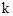is

The principle of linear superposition holds in this case. The displacement can be calculated for an individual force. Then the total displacement is the sum of the displacements for the individual forces.

Assume that the only force acting on the system is at degree-of-freedom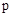. The modal displacement at degree-of-freedomis

The physical displacement at degree-of-freedom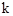for the single force case is

The receptance function for the single force applied becomes

The numerator in the series shows the relationship between the receptance function and the mass-normalized eigenvector terms.

Two-Degree-of-Freedom System, Frequency Domain Example

The system in has the parameters previously shown in Table 6.1, with 5% damping for each mode. Its natural frequencies are again 20.0 and 42.5 Hz. Its receptance frequency response function from equation (1.31) is given in Figure 1.11 for four permutations. The first index in the legend is the response location. The second is the applied force location, where each location is a degree-of-freedom. The phase angles could also be plotted but are omitted for brevity.

SECTION 3
Base Excitation
SDOF Response Equations

This case is also referred to as support motion or seismic excitation. The base excitation model and its free-body diagram are

The base displacement is y, and base velocity is. The equation of motion for base excitation is derived using Newton’s law.

The challenge with equation (1.34) is that the base displacement and base velocity are usually unknown, but the base acceleration is known. The workaround is to define a relative displacement z as

Through substitution and algebraic manipulation, the following ordinary differential equation is derived in terms of the relative displacement.

The resulting equation of motion is non-homogenous. Theterm on the right-hand side can be regarded as a virtual force.

The equation for base excitation can be further simplified as

The absolute acceleration is then calculated as

Equation (1.37) can be solved via Laplace transforms if the base acceleration is deterministic such as a sine function. A convolution integral is needed if the excitation varies arbitrarily with time. The resulting relative displacement equation for acceleration base excitation is

Equation (1.37) can also be solved in the frequency domain. Time and frequency domain analyses should yield the same results per Parseval's theorem.

The relative displacement convolution integral has an embedded impulse response function.

The Laplace transform of the impulse response function is the steady-state transmissibility function for relative displacement.

This transmissibility function can be represented in the frequency domain by setting, where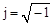.

The relative displacement transmissibility function in equation (1.42) is the Fourier transform of the impulse response function in equation (1.40). Fourier transforms are covered in Section 15.

The absolute acceleration response convolution integral is

The convolution integral in equation (1.43) is computationally inefficient, however. An alternative is to use the Smallwood ramp invariant digital recursive filtering relationship . The recursive filtering algorithm is fast and is the numerical engine used in almost all shock response spectrum software. It is also accurate assuming that the data has a sufficiently high sample rate and is free from aliasing. The algorithm is discussed further in Section 18.5.

The embedded impulse response function in equation (1.43) is

The Laplace transform of the impulse response function is the steady-state transmissibility function for absolute acceleration.

The transmissibility function in the frequency domain is

Input & Response Example, Base Excitation

The single-degree-of-freedom system in Figure 1.12 has a natural frequency of 10 Hz and an amplification factor Q=10 for this example. It is excited into resonance by a 1 G sinusoidal base excitation at 10 Hz. The exact response can be calculated via a Laplace transform solution. The result is shown in Figure 1.13.

The response calculation could be repeated for numerous frequency and amplification factor cases to determine the maximum steady-state response for each case. The transmissibility results for three amplification factor cases are shown in Figure 1.14. The frequency ratio is the excitation frequency f divided by the natural frequency fn. The magnitude is the steady response divided by the input amplitude. The transmissibility tends to unity gain when the excitation frequency is much less than the natural frequency. Resonant amplification occurs when the frequency ratio is at or very near one. Isolation occurs when the excitation frequency is greater than √2 times the natural frequency.

Note that the Q value is approximately equal to the peak transmissibility at resonance in Figure 1.14. Also, the resonant time domain response in Figure 12.13 reached a steady-state peak of Q times the base input. This suggests that sine vibration testing could be a way for measuring the amplification factor and its corresponding damping ratio by noting the peak response either in the frequency or time domain. But this simple relationship should be considered only for the special case of a single-degree-of-freedom system. Multi-degree-of-freedom systems and continuous systems have responses which depend on damping as well as on mode shape parameters.

Consider a cantilever beam subjected to base excitation exciting its fundamental bending mode. An accelerometer is mounted on the beam’s free end, and another at its midpoint. The peak response at free end will be higher than that at the midpoint due to the respective location-dependent modal gain factors. The half-power bandwidth method is a much more reliable damping identification method for measured response data from this beam and from general structures. This method would yield the same damping value from either of the two accelerometer data results. See the examples in Sections 13.4 and 13.5.

The transmissibility function plotted in Figure 1.14 was introduced in Section 12.3. The magnitude of H(ρ) can be represented as

The phase angle by which the response lags the input is

Alternate Base Excitation Method using Seismic Mass

Recall the semi-definite system in Section 6.1.6 and the two-DOF system subjected to an applied force in Section 12.2.6. A hybrid of these systems can be configured for base excitation by setting the bottom mass to a value vastly larger than the top mass by a factor of, say, 1e+04. Then an external force is applied to the base to produce the desired acceleration. This approach is unnecessary for SDOF system base excitation but becomes very useful for complex, multi-degree-of-freedom systems. The equation of motion is

The hybrid equation is solved using the methods in Section 12.2.6. There is a rigid-body mode at zero frequency. The second modal frequency is

The second modal frequency becomes

The second frequency is essentially equal to the top mass and the spring as a single-degree-of-freedom system.

Repeat the example in Section 12.3.2. The natural frequency was 10 Hz in this example with Q=10. Use of the seismic mass method requires explicit knowledge of the mass and stiffness values, as shown for this example in Table 12.3. The top mass is excited into resonance by a 1 G sinusoidal base excitation at 10 Hz via an equivalent 100,000 lbf force applied to the bottom, seismic mass. The acceleration response is shown in Figure 12.16. The result is the same as that in Figure 12.13 with the response time histories in both plots reaching 10 G steady-state.

Table 3.1. Seismic Mass, Hybrid System, Parameters
Variable Value1.0e+05 lbm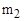10 lbm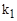102.2 lbf/in

Note that in some cases it may be necessary to ground the seismic mass with a very soft spring for numerical stability purposes, such that the fundamental frequency is just slightly above the zero frequency but is still much less than the frequency in equation (1.51).

Two-DOF Response Equations, Time Domain

Consider the system in the above figure, which is sometimes referred to as two-stage isolation per Reference . The equation of motion can be derived using Newton’s law.

The familiar challenge with equation (1.52) is that the base displacement and base velocity are usually unknown, but the base acceleration is known. The workaround is to define a relative displacement such that

Substitution of the relative displacements into equation (1.52) yields the following equation after algebraic manipulation.

The natural frequencies and modes shapes are calculated using the method from Section 6.1.2. The modal decoupling process is essentially the same as that in Section 12.2.6, with force vector changed to

The relative displacement is represented in terms of the mass-normalized eigenvector matrix and the modal displacement.

The uncoupled equations of motion are

The modal displacement can be calculated via Laplace transforms for a deterministic base acceleration. Modal accelerations can be calculated by twice differentiating the modal displacements.

A ramp invariant digital filtering relationship can be used for a base excitation which varies arbitrarily with time. Such equations are available for direct calculation of both the modal displacement and acceleration. The filtering equations are taken from Reference  given the virtual force and displacement characteristics of equation (1.57), rather than the SDOF formulas for base excitation given in References , .

The physical relative displacement can then be calculated by applying the modal displacements to equation (1.56). The physical relative acceleration can then be determined from the modal acceleration via the same eigenvector matrix transformation in equation (1.56). The physical absolute acceleration is then found by adding the base acceleration to the physical relative acceleration.

Two-DOF Response Equations, Time Domain Example

The system in Figure 1.17 has the parameters previously shown in Table 6.1 with 5% damping for each mode. Its natural frequencies are again 20.0 and 42.5 Hz. It is subjected to sinusoidal base excitation, with zero initial conditions. The base acceleration amplitude is 1 G with frequency at 20.0 Hz, which is the same as the fundamental frequency. The results are shown in Figure 1.18. The analysis is repeated with the excitation frequency at 42.5 Hz, as shown in Figure 1.19.Figure 3.7. Acceleration Responses to 20.0 Hz ExcitationFigure 3.8. Acceleration Responses to 42.5 Hz Excitation The responses are 180 degrees out-of-phase with one another.
Two-DOF Response Equations, Frequency Domain

Equation (1.57) can be used in the frequency domain for the case of steady-state harmonic base excitation. Define base acceleration and modal displacement coordinates as follows

Substitute these harmonic terms into Equation (1.57). The result for modal displacement after simplification is

The physical relative displacements are then found from

The physical relative displacement at degree-of-freedomis

The transmissibility function relating physical relative displacement to base excitation is

The transmissibility function relating physical relative acceleration to base excitation is